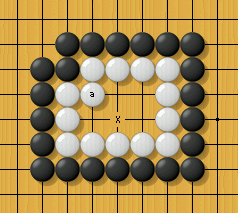HomeMadLabInfoLambda-orderLambda-order
 Lambda-order is a concept related to the lambda-search algorithm. In my paper on this algorithm, you can find formal definitions and a lot more information, but to give you an idea of the concept, consider the following position: Black to capture 'a'Can black capture 'a? The answer is yes: White is captured in a simple ladder as follows: Ladder: lambda-order n=1Note that after each black move White is in atari (i.e. under a direct threat of being captured). After each white move, White is no longer in atari. After Black 5, White is doomed. In Go, this is called a ladder, and in the lambda-search algorithm used in MadLab such a problem has lambda-order n=1. This concept of threats and moving out of threats can be generalized. Consider the following position: Black to capture 'a'Now Black can no longer play an atari on the white stone. But he can still capture 'a'. This goes as follows: Loose ladder: lambda-order n=2Note that after each black move Black threatens to capture White in a ladder if White does not answer. After each white move, White can no longer be caught in a ladder. No matter what White plays after Black 5, White cannot avoid being caught in a subsequent ladder. In Go, such a problem is called a loose ladder, and in the lambda-search terminology it is said to have  lambda-order n=2. This concept extends to other kinds of goals than capturing a single block of stones (e.g., double-goal capture problems, connection problems etc.). Lambda-orders up to n=3 or n=4 ("loose loose ladders" and "loose loose loose ladders") are quite common in open-space tesuji problems, whereas problems with lambda-order n=5 or more often have a somewhat different character (such problems typically have tsume-go (life & death) or semeai flavour). So MadLab usually prefers problems with lambda-order up to and including n=4, even though it can solve (some) problems of order 5 or higher. Enclosed problems can have very high lambda-orders. As an example the well-known nakade shape has lambda-order n=7. Black to capture "a" (lambda-order n=7)This means that the attacker must spend n+1 = 8 extra moves on removing the white block (starting at Black "x"). This corresponds to White having 8 effective liberties as opposed to the 5 "physical" ones. MadLab does solve this problem. But if Black had had less liberties (7 or less to be precise), White would have had time to stage a counter-attack on the black stones, and thus it would be a much more complicated (semeai) problem. Note that regarding single-goals captures there is a relationship between the number of liberties of the block to capture and the lambda-order: the lambda-order to kill the stones is at least libs - 1, where libs is the number of liberties of the block. So if a block has 5 liberties, it takes at least a lambda-search of order 4 to kill it. So there is a correlation between lambda-order and the number of liberties, but it is not a strict relationship. For instance, in the above figure White has 5 liberties, meaning that the problem could be solved in a search of lambda-order n=4. However, the lambda-order actually is as high as n=7. How do problems with lambda-order n=3 look like? The following three problems are all of order n=3: As stated above, the concept of lambda-order is not restricted to single-goal capture tesuji's. The following gives an example of a double-threat tesuji with lambda-order n=2 (loose ladder): Example 2 (n=2, double-threat)